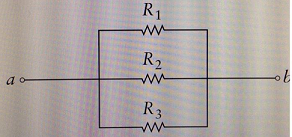# A potential difference of 3.7 V is applied between points a and b in the figure below. Suppose...

## Question:

A potential difference of 3.7 V is applied between points a and b in the figure below.

Suppose that {eq}R_1 = 6.0\ \Omega {/eq}, {eq}R_2 = 14\ \Omega {/eq} , {eq}R_3 = 6.0\ \Omega {/eq}

(1)Find the current through resistor {eq}R_1 {/eq}.

Express your answer using two significant figures.

Answer in A

(2)Find the current through resistor {eq}R_2 {/eq}

Express your answer using two significant figures.

Answer in A## Application of Ohm's Law:

When various resistors are connected in parallel combination to a battery, all the resistors get the voltage of battery(V). Therefore, the current(I) through any resistance(R) can be individually found by applying ohm's law as:

• {eq}V = R\times I {/eq}

## Answer and Explanation:

Given Data:

• Three resistors in parallel combination: {eq}\\R_1 = 6.0\ \Omega\\R_2 = 14.0\ \Omega\\R_3 = 6.0\ \Omega{/eq}
• Battery voltage applied between a and b, V = 3.7 V

(1)Finding current {eq}(I_1){/eq} through resistor {eq}R_1{/eq}

Applying Ohm's Law:

• {eq}I_1 = \dfrac{V}{R_1}{/eq}
• {eq}I_1 = \dfrac{3.7}{6} = 0.62\ A{/eq}

(2)Finding current {eq}(I_2){/eq} through resistor {eq}R_2{/eq}

Similarly applying ohm's law:

• {eq}I_2 = \dfrac{V}{R_2}{/eq}
• {eq}I_2 = \dfrac{3.7}{14} = 0.26\ A{/eq}

#### Learn more about this topic:Ohm's Law: Definition & Relationship Between Voltage, Current & Resistance

from CLEP Natural Sciences: Study Guide & Test Prep

Chapter 6 / Lesson 9
417K